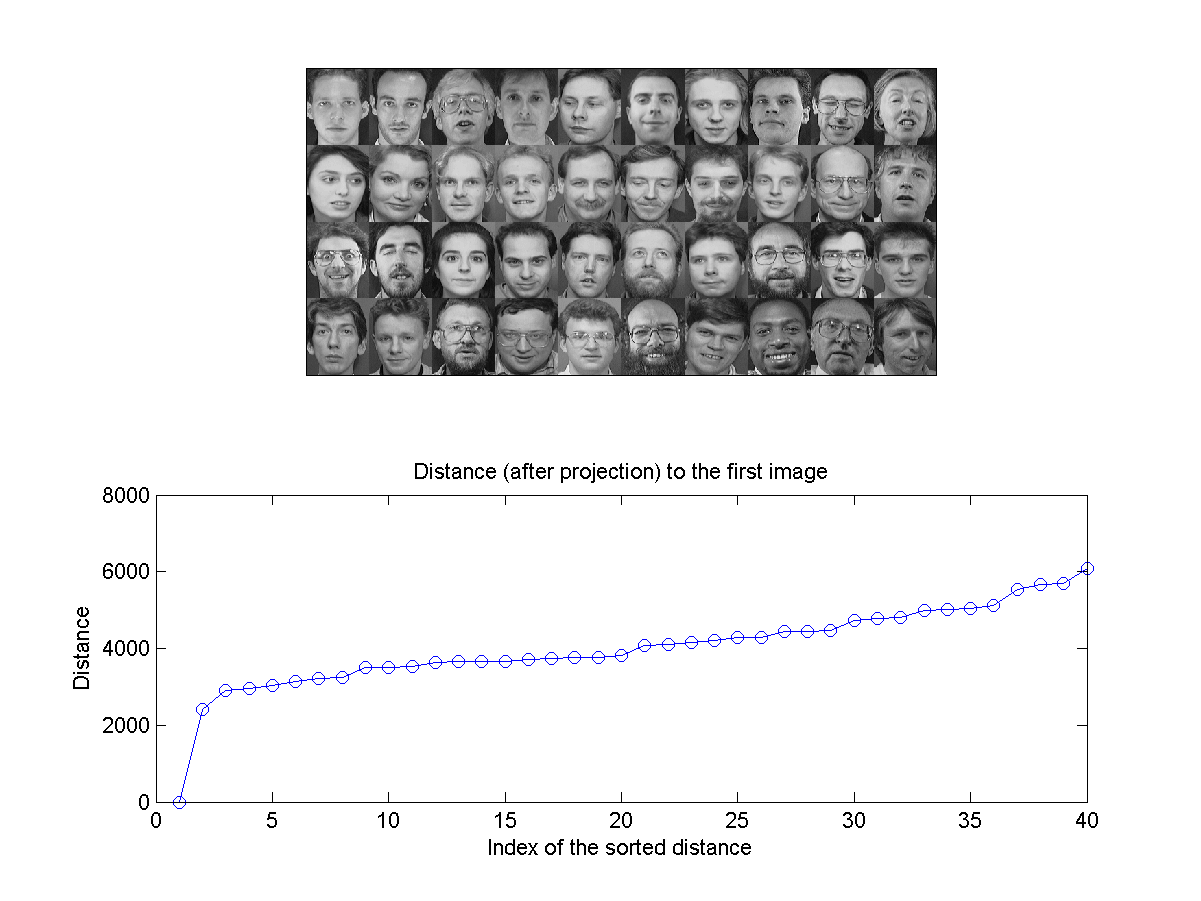11-4 PCA for Face Recognition

This section explains the use of PCA for face recognition.

First of all, you need to read the face dataset using the following script:

Example 1: faceRecog/faceDataRead01.mimageDir=[mltRoot, '/dataSet/att_faces']; faceData=recursiveFileList(imageDir, 'pgm'); fprintf('Reading %d face images from %s...', length(faceData), imageDir); tic for i=1:length(faceData) % fprintf('%d/%d: file=%s\n', i, length(faceData), faceData(i).path); faceData(i).image=imread(faceData(i).path); end fprintf(' ===> %.2f sec\n', toc); fprintf('Saving faceData.mat...\n'); save faceData faceDataReading 400 face images from D:\users\jang\matlab\toolbox\machineLearning/dataSet/att_faces... ===> 0.98 sec Saving faceData.mat...

The face data is then save as a structure array faceData of size 400 in faceData.mat.

You can display one of the face image as follows:

Example 2: faceRecog/faceDisplay01.mload faceData.mat subplot(1,2,1); imagesc(faceData(1).image); colormap(gray) axis image; subplot(1,2,2); surf(double(faceData(1).image)); colormap(gray) axis image; shading interp; view(140, 80);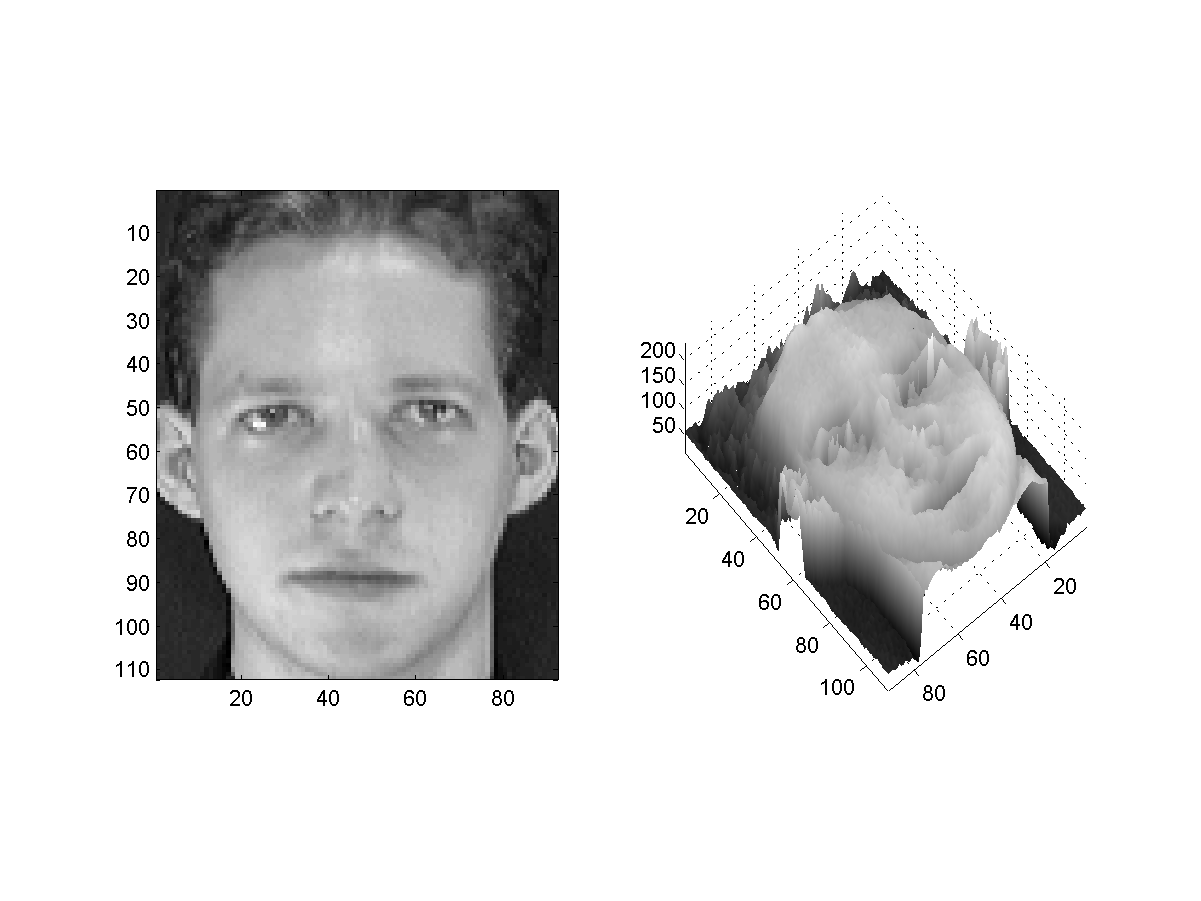If you want to display face images of the first 4 persons, use "montage" to do so:

Example 3: faceRecog/faceDisplay02.mload faceData.mat filePaths={faceData.path}; montage(filePaths(1:40), 'Size', [nan, 10]);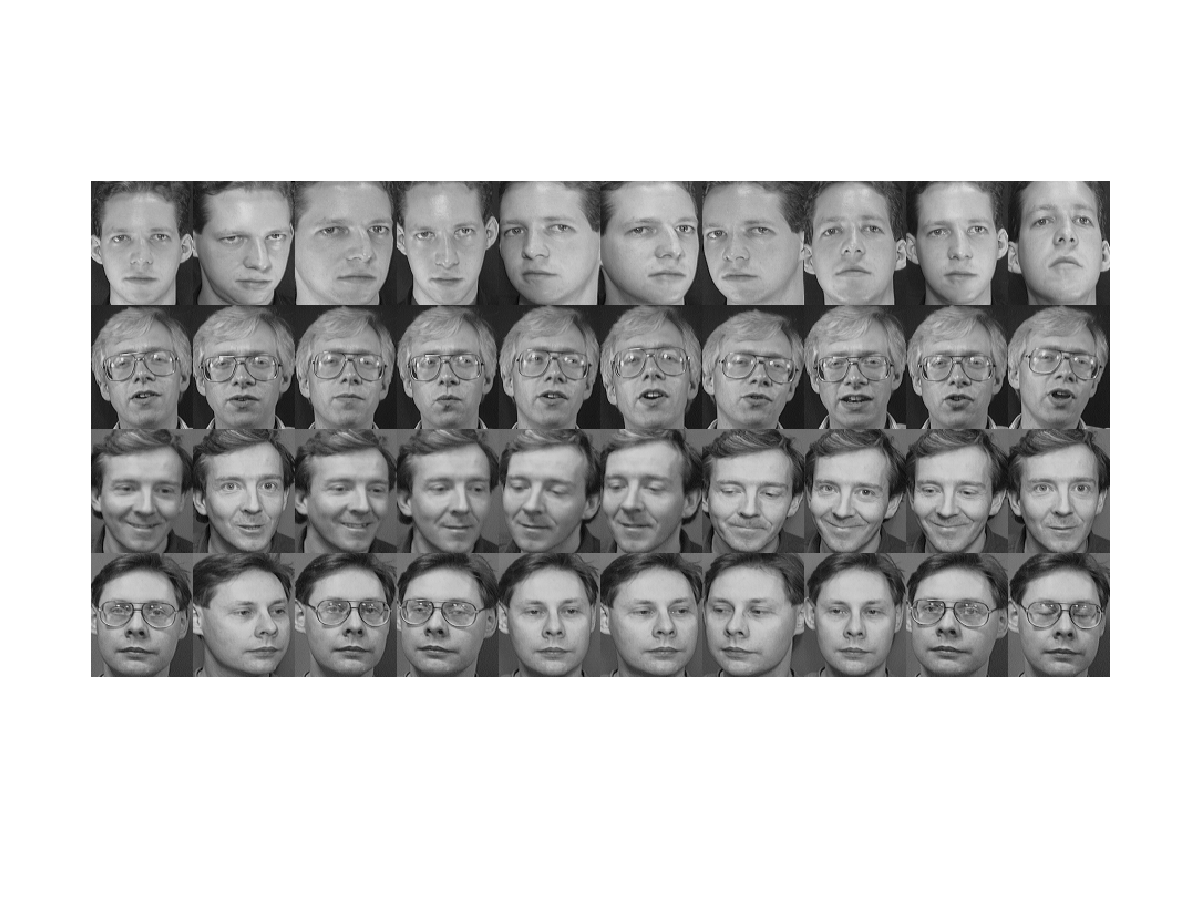Alternatively, you can display all the images as a big one:

Example 4: faceRecog/faceDisplay03.mload faceData.mat filePaths={faceData.path}; montage(filePaths, 'Size', [nan, 30]); [Warning: Image is too big to fit on screen; displaying at 33%] [> In <a href="matlab: opentoline('C:\Program Files\MATLAB\R2013a\toolbox\images\imuitools\private\initSize.m',72,1)">imuitools\private\initSize at 72</a> In <a href="matlab: opentoline('C:\Program Files\MATLAB\R2013a\toolbox\images\imuitools\imshow.m',283,1)">imshow at 283</a> In <a href="matlab: opentoline('C:\Program Files\MATLAB\R2013a\toolbox\images\imuitools\montage.m',137,1)">montage at 137</a> In <a href="matlab: opentoline('D:\users\jang\books\dcpr\example\faceRecog\faceDisplay03.m',3,1)">faceDisplay03 at 3</a> In <a href="matlab: opentoline('D:\users\jang\books\goWriteOutputFile.m',83,1)">goWriteOutputFile>dummyFunction at 83</a> In <a href="matlab: opentoline('D:\users\jang\books\goWriteOutputFile.m',53,1)">goWriteOutputFile at 53</a>]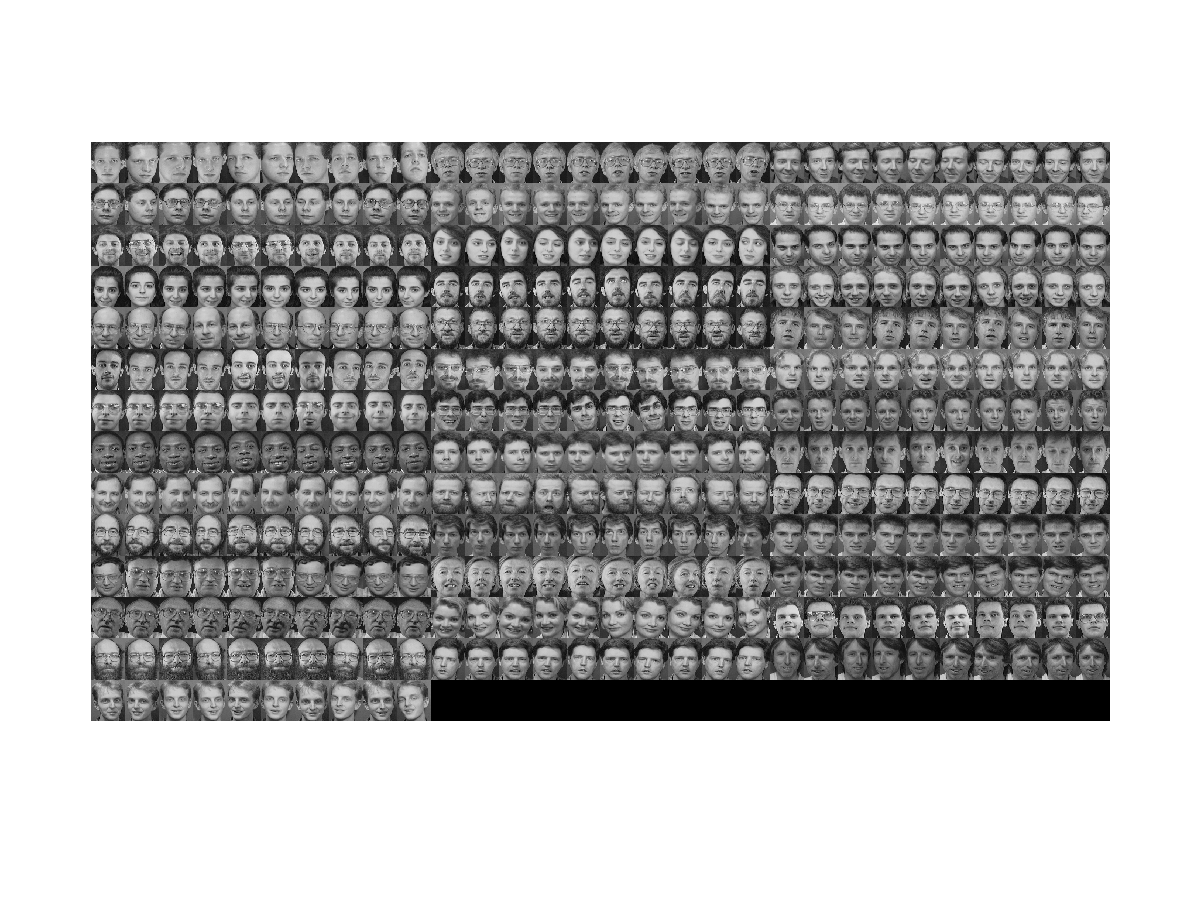To try PCA on these face images, we need to find the mean face first:

Example 5: faceRecog/meanFaceDisplay01.mload faceData.mat allFaces=double(cat(3, faceData.image)); meanFace=mean(allFaces, 3); imagesc(meanFace); axis image; colormap(gray);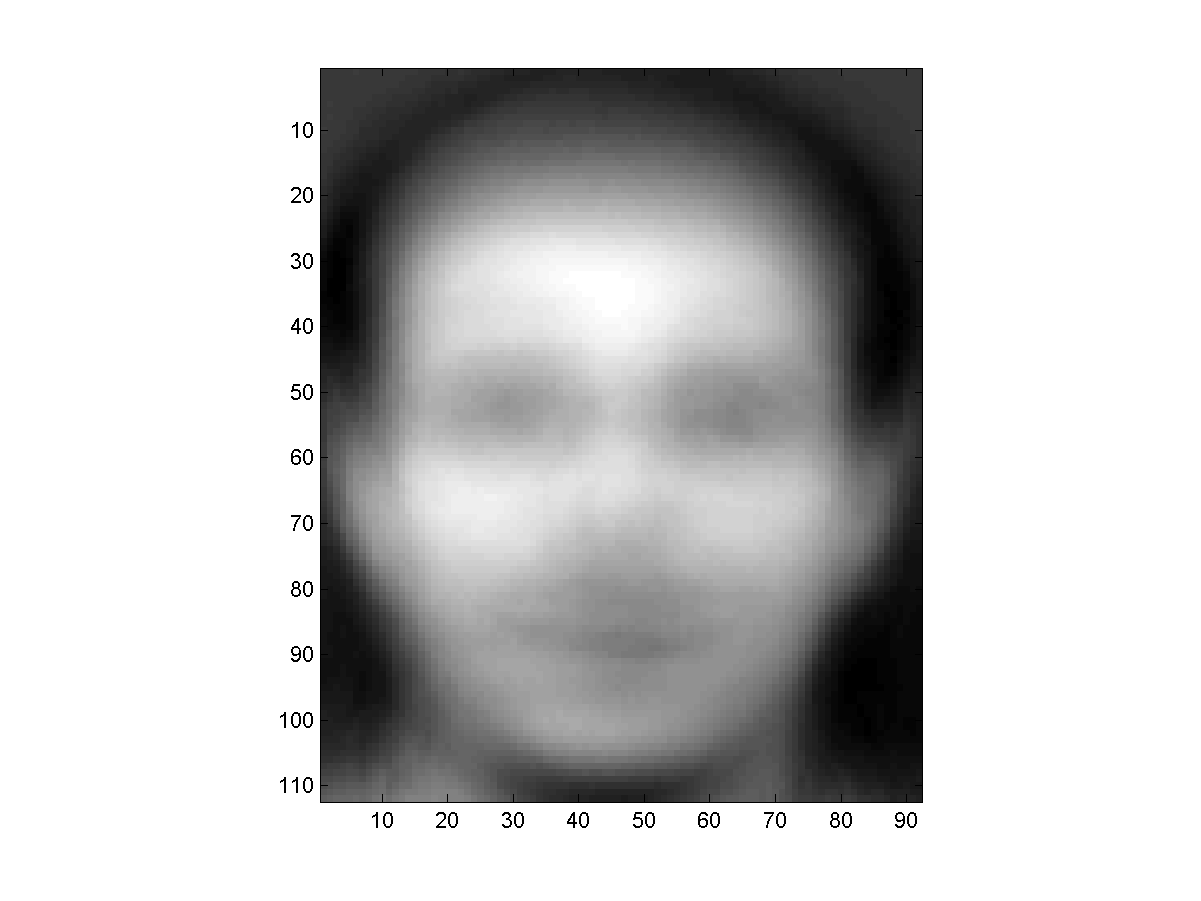Now we are ready to put all face images (after mean subtraction) into a big matrix A and find eigenvalues and eigenvectors of A*A' for PCA analysis. In particular, we can plot the percentage of total variance versus number of eigenvalues to get an idea as how PCA can "squeeze" the variance into the first few eigenvalues, as follows.

Example 6: faceRecog/varVsPcaEigNum01.mload faceData.mat [rowDim, colDim]=size(faceData(1).image); % ====== Compute mean face meanFace=mean(double(cat(3, faceData.image)), 3); % ====== Put all face into a big matrix fprintf('Put all images into a big matrix... '); tic for i=1:length(faceData) A(:,i)=double(faceData(i).image(:))-meanFace(:); end fprintf(' ===> %.2f sec\n', toc); % ====== Perform PCA fprintf('Perform PCA... '); tic [A2, eigVec, eigValue]=pca(A); fprintf(' ===> %.2f sec\n', toc); % ====== Plot variance percentage vs. no. of eigenvalues cumVar=cumsum(eigValue); cumVarPercent=cumVar/cumVar(end)*100; plot(cumVarPercent, '.-'); xlabel('No. of eigenvalues'); ylabel('Cumulated variance percentage (%)'); title('Variance percentage vs. no. of eigenvalues'); fprintf('Saving results into eigenFaceResult.mat...\n'); save eigenFaceResult A2 eigVec cumVarPercent rowDim colDim Put all images into a big matrix... ===> 0.04 sec Perform PCA... ===> 0.31 sec Saving results into eigenFaceResult.mat...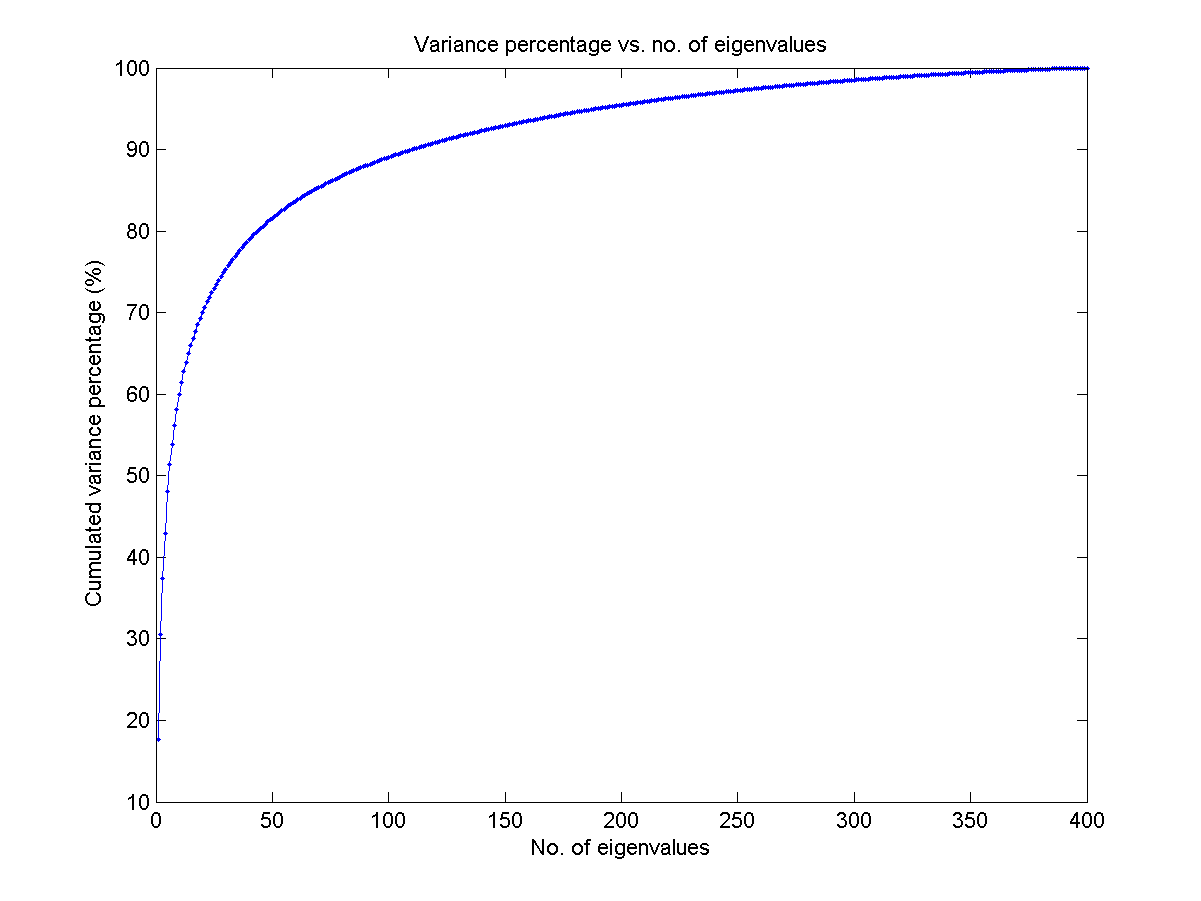Once we have the eigenvectors of A*A', we can display the first few eigenfaces:

Example 7: faceRecog/eigenFaceDisplay01.mload eigenFaceResult.mat % load A2, eigVec, rowDim, colDim, etc reducedDim=16; % Display the first 16 eigenfaces eigenfaces = reshape(eigVec, rowDim, colDim, size(A2,2)); side=ceil(sqrt(reducedDim)); for i=1:reducedDim subplot(side,side,i); imagesc(eigenfaces(:,:,i)); axis image; colormap(gray); set(gca, 'xticklabel', ''); set(gca, 'yticklabel', ''); end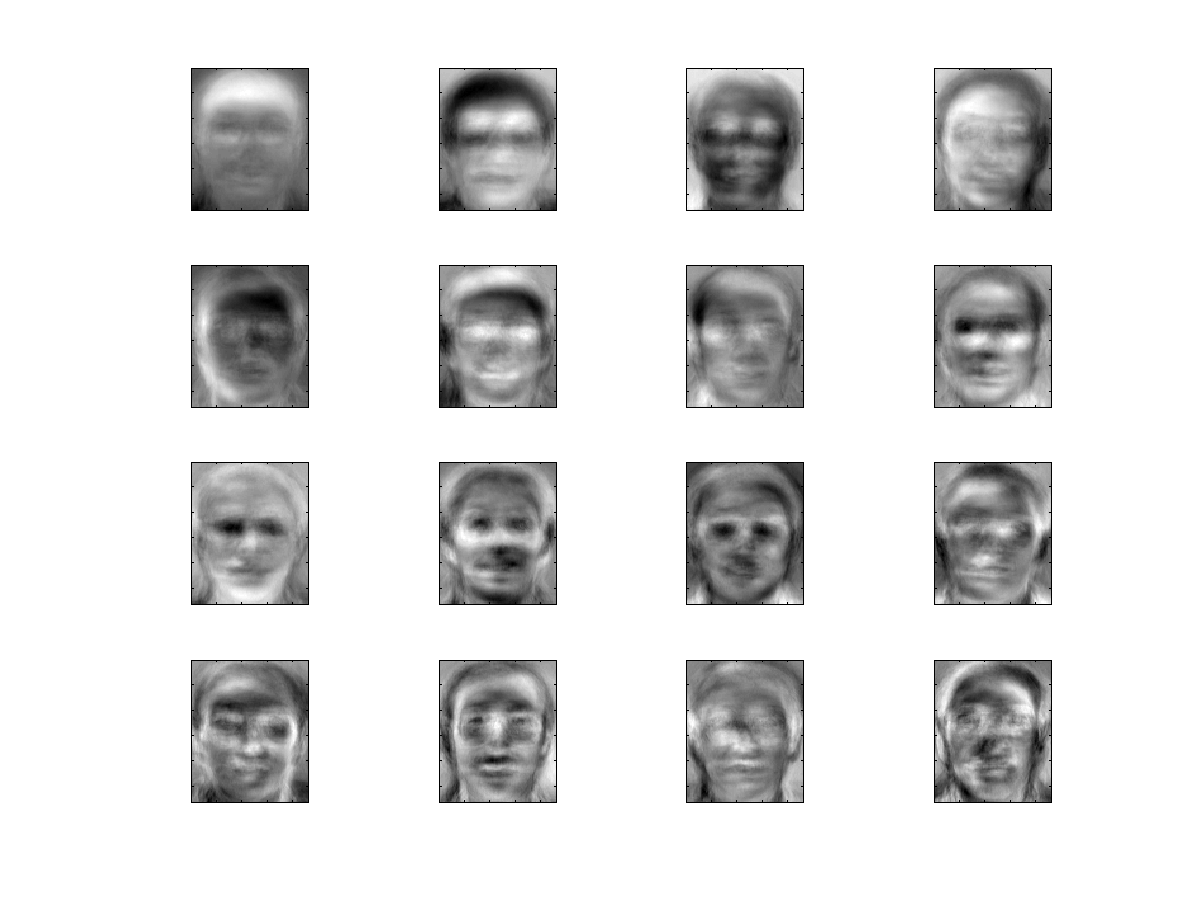For purpose of visualization, we can project the original faces into 2D face space:

Example 8: faceRecog/face2dPcaProj01.mload faceData.mat load eigenFaceResult.mat % Load A2, eigVec, rowDim, colDim, etc DS.input=A2(1:2,:); DS.outputName=unique({faceData.parentDir}); DS.output=zeros(1, size(DS.input,2)); for i=1:length(DS.output) DS.output(i)=find(strcmp(DS.outputName, faceData(i).parentDir)); DS.annotation{i}=faceData(i).path; end dsScatterPlot(DS); [recogRate, computed, nearestIndex]=knncLoo(DS); fprintf('Recog. rate = %.2f%%\n', 100*recogRate); Recog. rate = 39.00%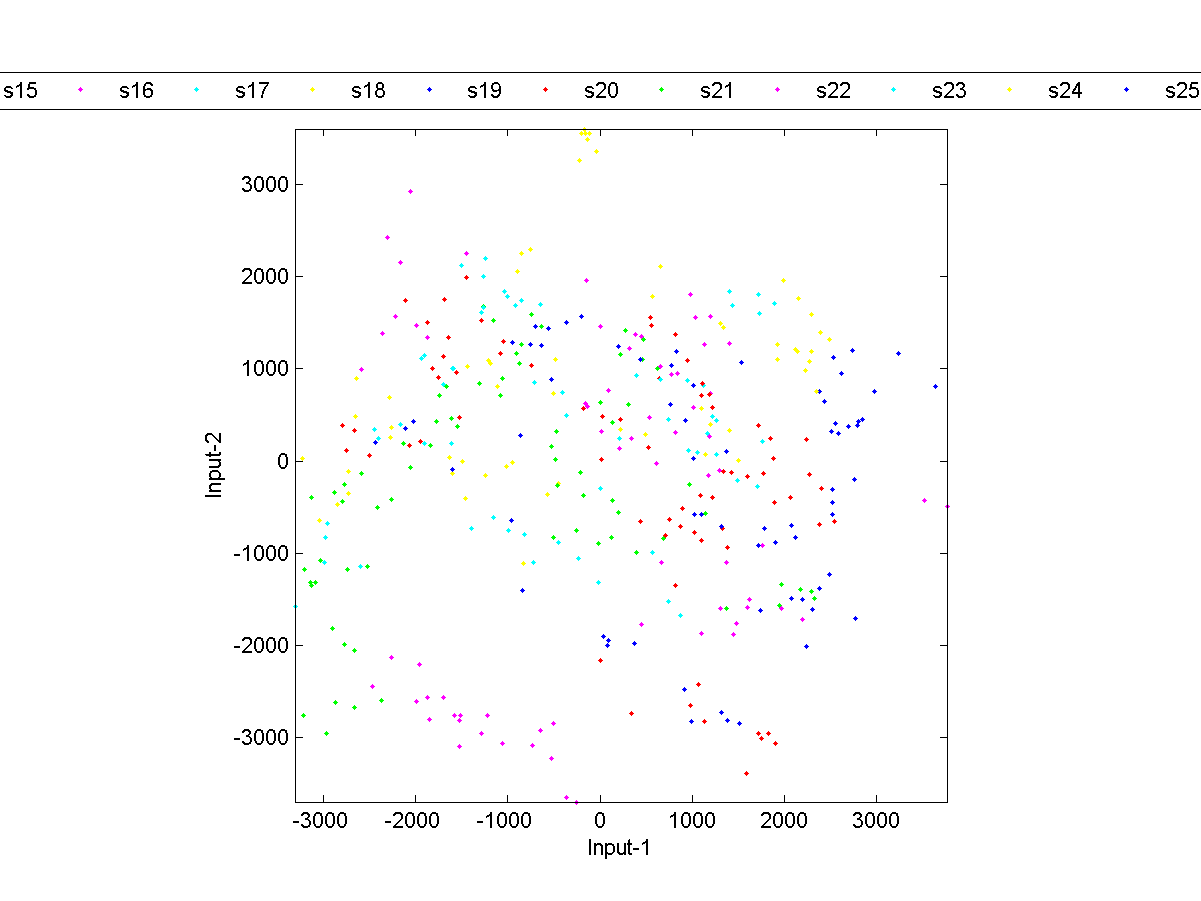The leave-one-out recognition rate of KNNC over the projected dataset is only 39.00%. This is a bit on the low side since the overall accounted variance of 2 eigenvalues is only about 30.52%.

To find the best number of eigenvalues, we can perform an exhaustive search:

Example 9: faceRecog/optPcaEigNum01.mload faceData.mat load eigenFaceResult.mat % Load A2, eigVec, cumVarPercent, rowDim, colDim % ====== Create DS DS.input=A2; DS.outputName=unique({faceData.parentDir}); DS.output=zeros(1, size(DS.input,2)); for i=1:length(DS.output) DS.output(i)=find(strcmp(DS.outputName, faceData(i).parentDir)); DS.annotation{i}=faceData(i).path; end % ====== RR w.r.t. no. of eigenvectors myTic=tic; maxDim=100; rr=pcaPerfViaKnncLoo(DS, maxDim, 1); plot(1:maxDim, cumVarPercent(1:maxDim), '.-', 1:maxDim, rr*100, '.-'); grid on xlabel('No. of eigenfaces'); ylabel('LOO recog. rate & cumulated variance percentage'); [maxValue, maxIndex]=max(rr); line(maxIndex, maxValue*100, 'marker', 'o', 'color', 'r'); legend('Cumulated variance percentage', 'LOO recog. rate', 'location', 'southeast'); fprintf('Optimum number of eigenvectors = %d, with recog. rate = %.2f%%\n', maxIndex, maxValue*100); toc(myTic) LOO recog. rate of KNNC using 1 dim = 36/400 = 9% LOO recog. rate of KNNC using 2 dim = 152/400 = 38% LOO recog. rate of KNNC using 3 dim = 291/400 = 72.75% LOO recog. rate of KNNC using 4 dim = 326/400 = 81.5% LOO recog. rate of KNNC using 5 dim = 342/400 = 85.5% LOO recog. rate of KNNC using 6 dim = 361/400 = 90.25% LOO recog. rate of KNNC using 7 dim = 378/400 = 94.5% LOO recog. rate of KNNC using 8 dim = 381/400 = 95.25% LOO recog. rate of KNNC using 9 dim = 385/400 = 96.25% LOO recog. rate of KNNC using 10 dim = 385/400 = 96.25% LOO recog. rate of KNNC using 11 dim = 390/400 = 97.5% LOO recog. rate of KNNC using 12 dim = 391/400 = 97.75% LOO recog. rate of KNNC using 13 dim = 391/400 = 97.75% LOO recog. rate of KNNC using 14 dim = 390/400 = 97.5% LOO recog. rate of KNNC using 15 dim = 390/400 = 97.5% LOO recog. rate of KNNC using 16 dim = 389/400 = 97.25% LOO recog. rate of KNNC using 17 dim = 390/400 = 97.5% LOO recog. rate of KNNC using 18 dim = 390/400 = 97.5% LOO recog. rate of KNNC using 19 dim = 392/400 = 98% LOO recog. rate of KNNC using 20 dim = 390/400 = 97.5% LOO recog. rate of KNNC using 21 dim = 391/400 = 97.75% LOO recog. rate of KNNC using 22 dim = 391/400 = 97.75% LOO recog. rate of KNNC using 23 dim = 391/400 = 97.75% LOO recog. rate of KNNC using 24 dim = 393/400 = 98.25% LOO recog. rate of KNNC using 25 dim = 393/400 = 98.25% LOO recog. rate of KNNC using 26 dim = 393/400 = 98.25% LOO recog. rate of KNNC using 27 dim = 394/400 = 98.5% LOO recog. rate of KNNC using 28 dim = 395/400 = 98.75% LOO recog. rate of KNNC using 29 dim = 394/400 = 98.5% LOO recog. rate of KNNC using 30 dim = 394/400 = 98.5% LOO recog. rate of KNNC using 31 dim = 394/400 = 98.5% LOO recog. rate of KNNC using 32 dim = 394/400 = 98.5% LOO recog. rate of KNNC using 33 dim = 394/400 = 98.5% LOO recog. rate of KNNC using 34 dim = 393/400 = 98.25% LOO recog. rate of KNNC using 35 dim = 394/400 = 98.5% LOO recog. rate of KNNC using 36 dim = 394/400 = 98.5% LOO recog. rate of KNNC using 37 dim = 394/400 = 98.5% LOO recog. rate of KNNC using 38 dim = 394/400 = 98.5% LOO recog. rate of KNNC using 39 dim = 393/400 = 98.25% LOO recog. rate of KNNC using 40 dim = 393/400 = 98.25% LOO recog. rate of KNNC using 41 dim = 393/400 = 98.25% LOO recog. rate of KNNC using 42 dim = 393/400 = 98.25% LOO recog. rate of KNNC using 43 dim = 393/400 = 98.25% LOO recog. rate of KNNC using 44 dim = 393/400 = 98.25% LOO recog. rate of KNNC using 45 dim = 393/400 = 98.25% LOO recog. rate of KNNC using 46 dim = 392/400 = 98% LOO recog. rate of KNNC using 47 dim = 392/400 = 98% LOO recog. rate of KNNC using 48 dim = 392/400 = 98% LOO recog. rate of KNNC using 49 dim = 393/400 = 98.25% LOO recog. rate of KNNC using 50 dim = 393/400 = 98.25% LOO recog. rate of KNNC using 51 dim = 392/400 = 98% LOO recog. rate of KNNC using 52 dim = 392/400 = 98% LOO recog. rate of KNNC using 53 dim = 393/400 = 98.25% LOO recog. rate of KNNC using 54 dim = 393/400 = 98.25% LOO recog. rate of KNNC using 55 dim = 393/400 = 98.25% LOO recog. rate of KNNC using 56 dim = 393/400 = 98.25% LOO recog. rate of KNNC using 57 dim = 392/400 = 98% LOO recog. rate of KNNC using 58 dim = 392/400 = 98% LOO recog. rate of KNNC using 59 dim = 392/400 = 98% LOO recog. rate of KNNC using 60 dim = 391/400 = 97.75% LOO recog. rate of KNNC using 61 dim = 392/400 = 98% LOO recog. rate of KNNC using 62 dim = 392/400 = 98% LOO recog. rate of KNNC using 63 dim = 392/400 = 98% LOO recog. rate of KNNC using 64 dim = 392/400 = 98% LOO recog. rate of KNNC using 65 dim = 392/400 = 98% LOO recog. rate of KNNC using 66 dim = 392/400 = 98% LOO recog. rate of KNNC using 67 dim = 392/400 = 98% LOO recog. rate of KNNC using 68 dim = 392/400 = 98% LOO recog. rate of KNNC using 69 dim = 392/400 = 98% LOO recog. rate of KNNC using 70 dim = 392/400 = 98% LOO recog. rate of KNNC using 71 dim = 392/400 = 98% LOO recog. rate of KNNC using 72 dim = 392/400 = 98% LOO recog. rate of KNNC using 73 dim = 392/400 = 98% LOO recog. rate of KNNC using 74 dim = 392/400 = 98% LOO recog. rate of KNNC using 75 dim = 392/400 = 98% LOO recog. rate of KNNC using 76 dim = 392/400 = 98% LOO recog. rate of KNNC using 77 dim = 392/400 = 98% LOO recog. rate of KNNC using 78 dim = 392/400 = 98% LOO recog. rate of KNNC using 79 dim = 392/400 = 98% LOO recog. rate of KNNC using 80 dim = 393/400 = 98.25% LOO recog. rate of KNNC using 81 dim = 393/400 = 98.25% LOO recog. rate of KNNC using 82 dim = 393/400 = 98.25% LOO recog. rate of KNNC using 83 dim = 393/400 = 98.25% LOO recog. rate of KNNC using 84 dim = 393/400 = 98.25% LOO recog. rate of KNNC using 85 dim = 393/400 = 98.25% LOO recog. rate of KNNC using 86 dim = 393/400 = 98.25% LOO recog. rate of KNNC using 87 dim = 393/400 = 98.25% LOO recog. rate of KNNC using 88 dim = 393/400 = 98.25% LOO recog. rate of KNNC using 89 dim = 393/400 = 98.25% LOO recog. rate of KNNC using 90 dim = 393/400 = 98.25% LOO recog. rate of KNNC using 91 dim = 393/400 = 98.25% LOO recog. rate of KNNC using 92 dim = 393/400 = 98.25% LOO recog. rate of KNNC using 93 dim = 393/400 = 98.25% LOO recog. rate of KNNC using 94 dim = 393/400 = 98.25% LOO recog. rate of KNNC using 95 dim = 393/400 = 98.25% LOO recog. rate of KNNC using 96 dim = 393/400 = 98.25% LOO recog. rate of KNNC using 97 dim = 393/400 = 98.25% LOO recog. rate of KNNC using 98 dim = 393/400 = 98.25% LOO recog. rate of KNNC using 99 dim = 393/400 = 98.25% LOO recog. rate of KNNC using 100 dim = 393/400 = 98.25% Optimum number of eigenvectors = 28, with recog. rate = 98.75% Elapsed time is 21.484721 seconds.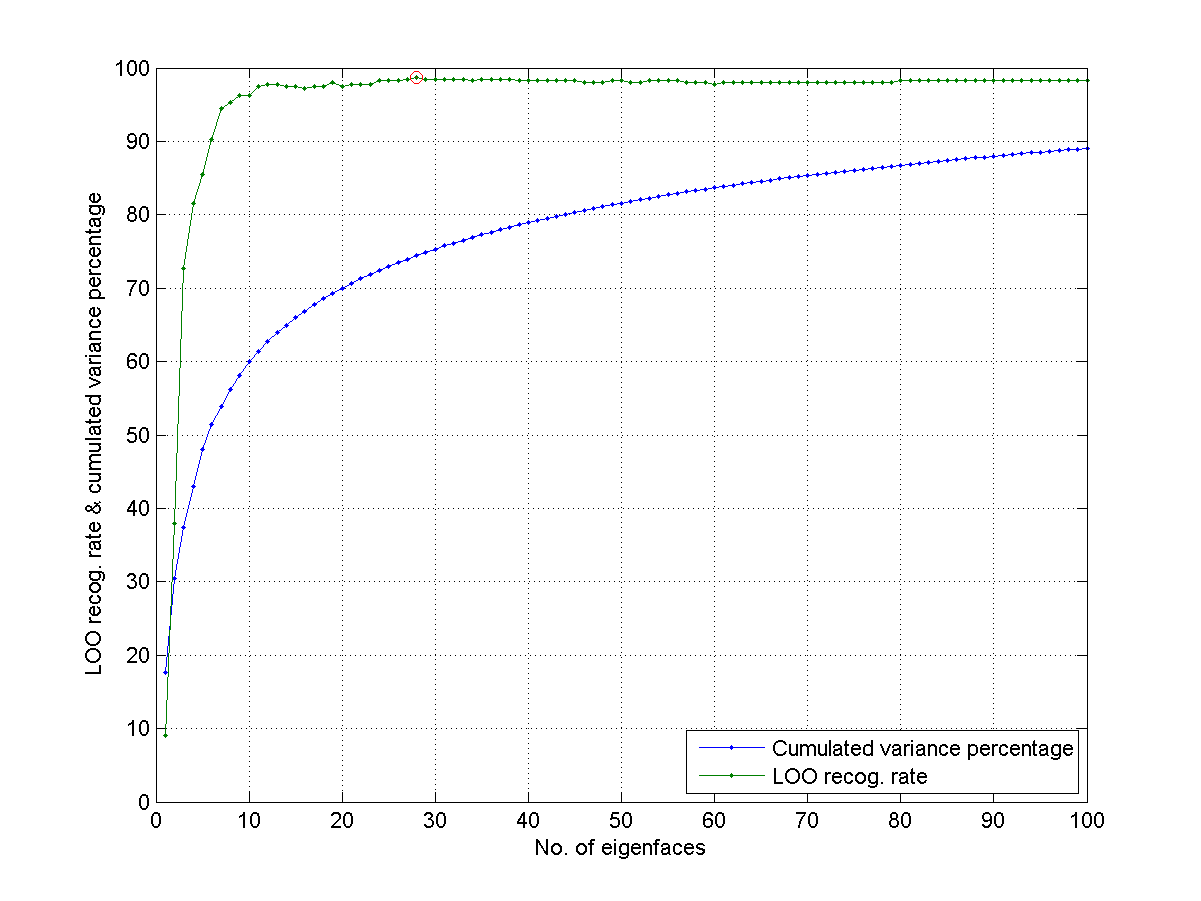Now it is obvious that the best recognition rate is 98.75%, which occurs when the number of used eigenvectors is 28, with a corresponding variance coverage of 74.44%. Under the given accuracy, we know there are 5 missclassified faces. The following example shows these misclassified faces, together with their 7 nearest neighbors:

Example 10: faceRecog/dispMisclassified01.mload faceData.mat load eigenFaceResult.mat % Load A2, eigVec, cumVarPercent, rowDim, colDim groundTruth=ones(10,1)*(1:40); groundTruth=groundTruth(:)'; % Create the groundtruth dim=28; % Take the first 28 eigenvectors A2=A2(1:dim, :); eigVec2=eigVec(:, 1:dim); % === Compute the nearest neighbors in the face space imageNum=size(A2,2); for i=1:imageNum query=A2(:,i); distance=distPairwise(query, A2); distance(i)=inf; [sortDistance, sortIndex]=sort(distance); misclassifiedData(i).nearestIndex=sortIndex; misclassifiedData(i).distance=sortDistance; misclassifiedData(i).miss=0; if groundTruth(i)~=groundTruth(sortIndex(1)) misclassifiedData(i).miss=1; end end missIndex=find([misclassifiedData.miss]); missNum=length(missIndex); n=7; % === Display the query and the nearest-neighbor faces for i=1:missNum faceIndex=missIndex(i); subplot(missNum, n+1, 1+(i-1)*(n+1)); imagesc(faceData(faceIndex).image); axis image title(sprintf('Query %d', i)); for j=1:n subplot(missNum, n+1, 1+j+(i-1)*(n+1)); imagesc(faceData(misclassifiedData(faceIndex).nearestIndex(j)).image); axis image title(sprintf('d=%.2f', misclassifiedData(faceIndex).distance(j))); end end colormap(gray); h=findobj(0, 'type', 'axes'); set(h, 'xticklabel', ''); set(h, 'yticklabel', '');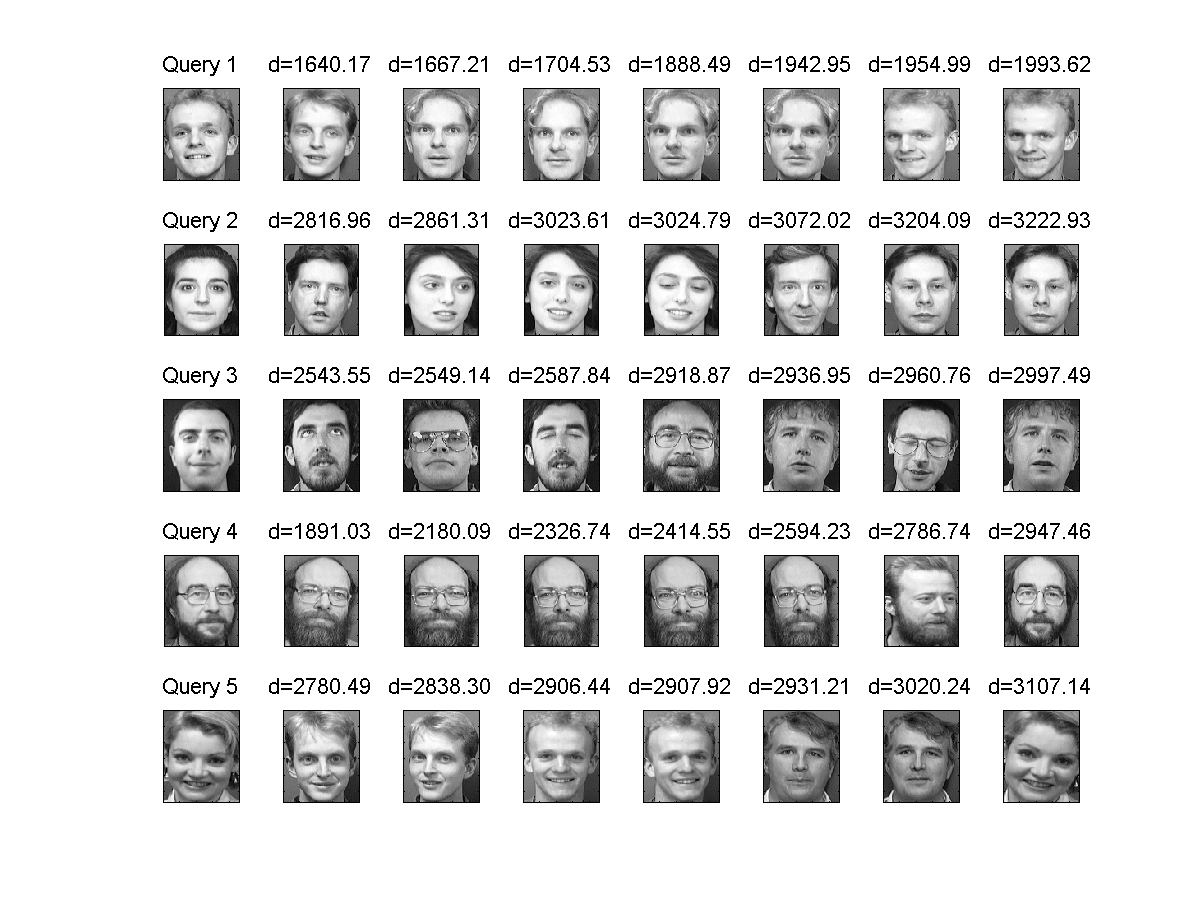However, it seems the retrieved faces do not resemble the query ones. It should be noted that the distance is based on the projected faces in the face space (spanned by the 28 eigenvectors corresponding to the top-28 eigenvalues). As a result, it should be more reasonable to show the query faces and the retrieved one in the face space, as shown next:

Example 11: faceRecog/dispMisclassified02.mload faceData.mat load eigenFaceResult.mat % Load A2, eigVec, cumVarPercent, rowDim, colDim groundTruth=ones(10,1)*(1:40); groundTruth=groundTruth(:)'; % Create the groundtruth dim=28; % Take the first 28 eigenvectors A2=A2(1:dim, :); eigVec2=eigVec(:, 1:dim); % === Compute the nearest neighbors in the face space imageNum=size(A2,2); for i=1:imageNum query=A2(:,i); distance=distPairwise(query, A2); distance(i)=inf; [sortDistance, sortIndex]=sort(distance); misclassifiedData(i).nearestIndex=sortIndex; misclassifiedData(i).distance=sortDistance; misclassifiedData(i).miss=0; if groundTruth(i)~=groundTruth(sortIndex(1)) misclassifiedData(i).miss=1; end end % === Create the projected face meanFace=mean(double(cat(3, faceData.image)), 3); meanFace=meanFace(:); for i=1:imageNum origFace=double(faceData(i).image); origFace=origFace(:); projFace=eigVec2*(eigVec2'*(origFace-meanFace))+meanFace; faceData(i).image2=reshape(projFace, rowDim, colDim); end missIndex=find([misclassifiedData.miss]); missNum=length(missIndex); n=7; % === Display the query and the nearest-neighbor faces for i=1:missNum faceIndex=missIndex(i); subplot(missNum, n+1, 1+(i-1)*(n+1)); imagesc(faceData(faceIndex).image2); axis image title(sprintf('Query %d', i)); for j=1:n subplot(missNum, n+1, 1+j+(i-1)*(n+1)); imagesc(faceData(misclassifiedData(faceIndex).nearestIndex(j)).image2); axis image title(sprintf('d=%.2f', misclassifiedData(faceIndex).distance(j))); end end colormap(gray); h=findobj(0, 'type', 'axes'); set(h, 'xticklabel', ''); set(h, 'yticklabel', '');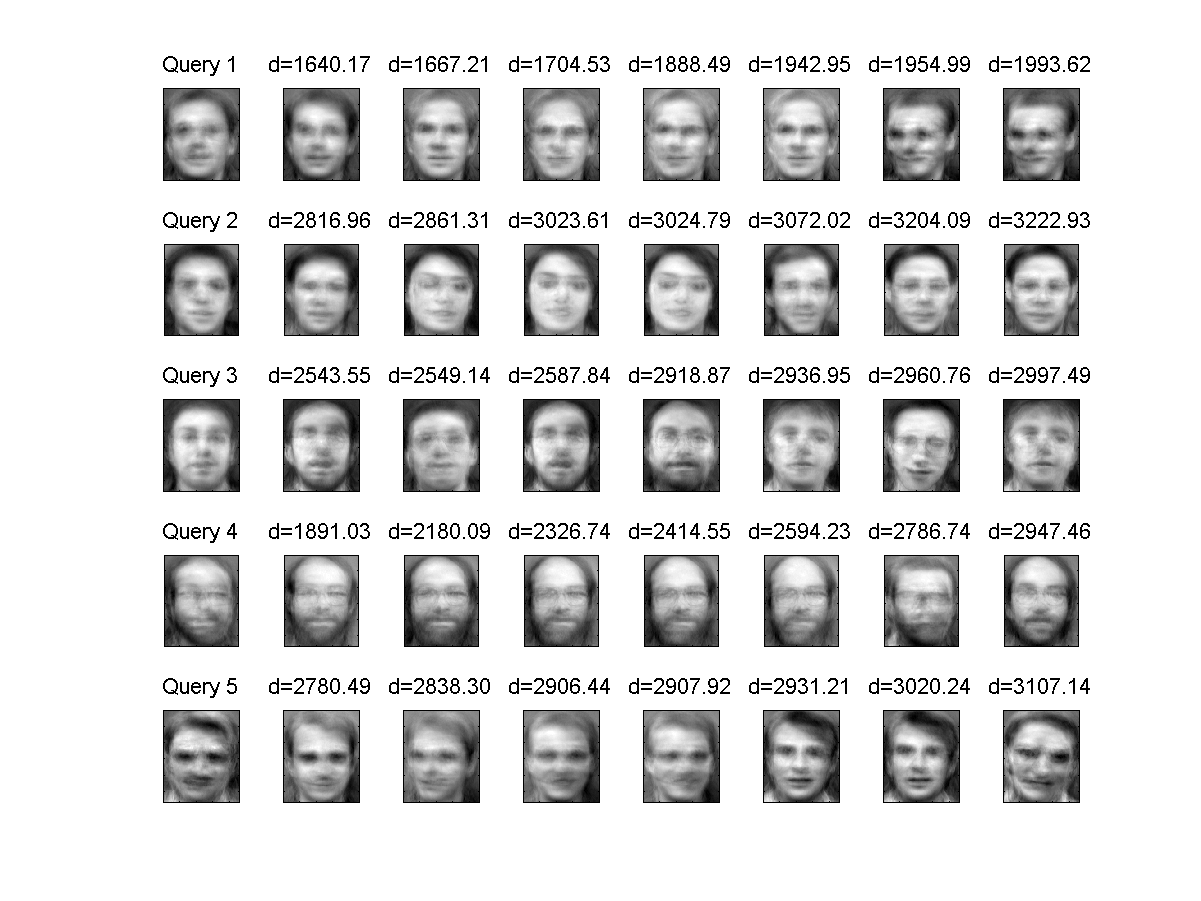However, the best recognition rate obtained above is overly optimistic since we used all faces for PCA projection when performing LOO test. A more objective way to estimate the recognition rate is to preclude the test data from PCA projection, as shown next. (Be warned that it takes a much longer time to run this example.)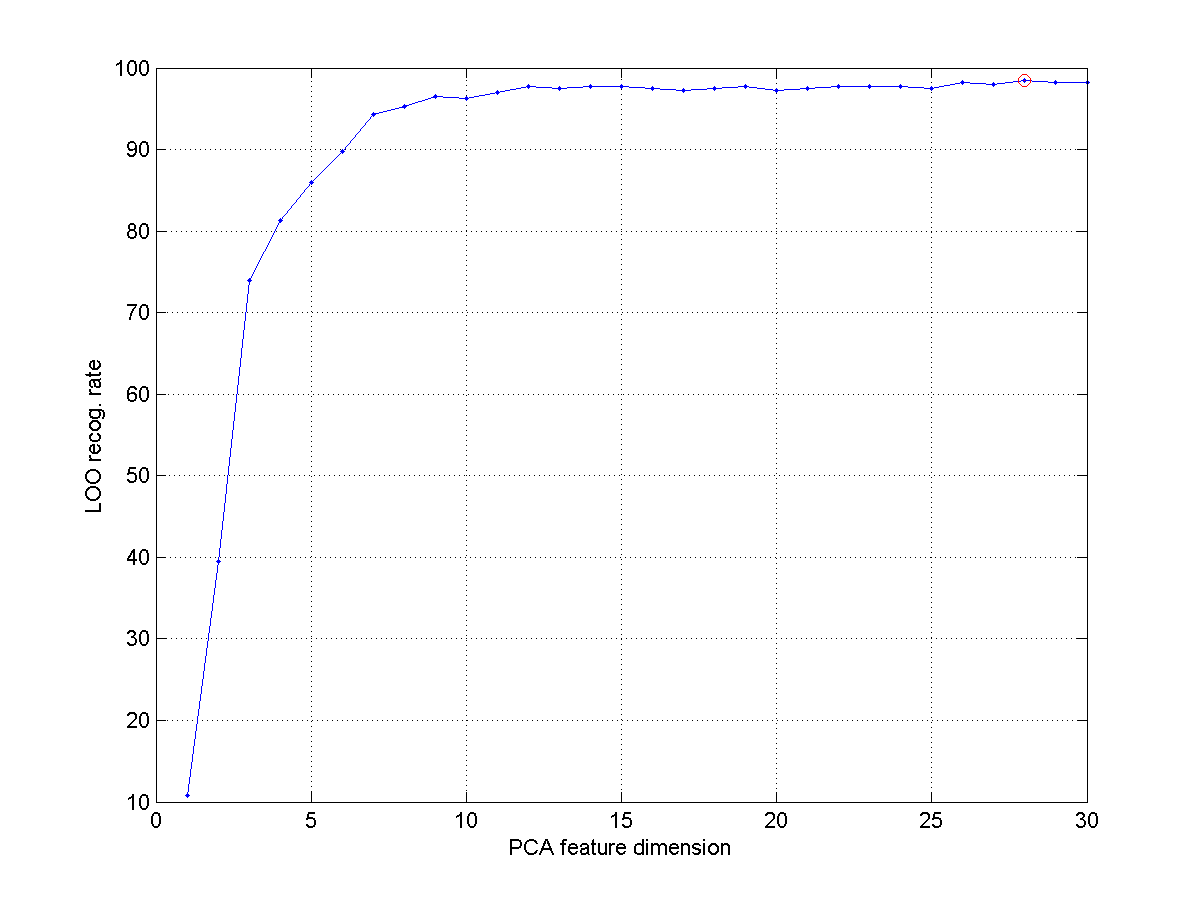From the above example, the more objective recognition rate is 98.50%, which occurs when the dimension for PCA projection is 28.

You may wonder what is the image after being projected onto the face space spanned by the optimum 28 eigenfaces. Here is an example to show the original and projected images, and their difference.

Example 13: faceRecog/facePcaProjDiff01.mload faceData.mat load eigenFaceResult.mat % Load A2, eigVec, cumVarPercent, rowDim, colDim eigVec2=eigVec(:, 1:28); % Take the first 28 eigenvectors origFace=double(faceData(31).image); origFace=origFace(:); meanFace=mean(double(cat(3, faceData.image)), 3); meanFace=meanFace(:); projFace=eigVec2*(eigVec2'*(origFace-meanFace))+meanFace; subplot(1,3,1); imagesc(reshape(origFace, rowDim, colDim)); axis image; colormap(gray); title('Original image'); subplot(1,3,2); imagesc(reshape(projFace, rowDim, colDim)); axis image; colormap(gray); title('Projected image'); subplot(1,3,3); imagesc(reshape(origFace-projFace, rowDim, colDim)); axis image; colormap(gray); title('Difference'); fprintf('Difference between orig. and projected images = %g\n', norm(origFace-projFace)); Difference between orig. and projected images = 1986.89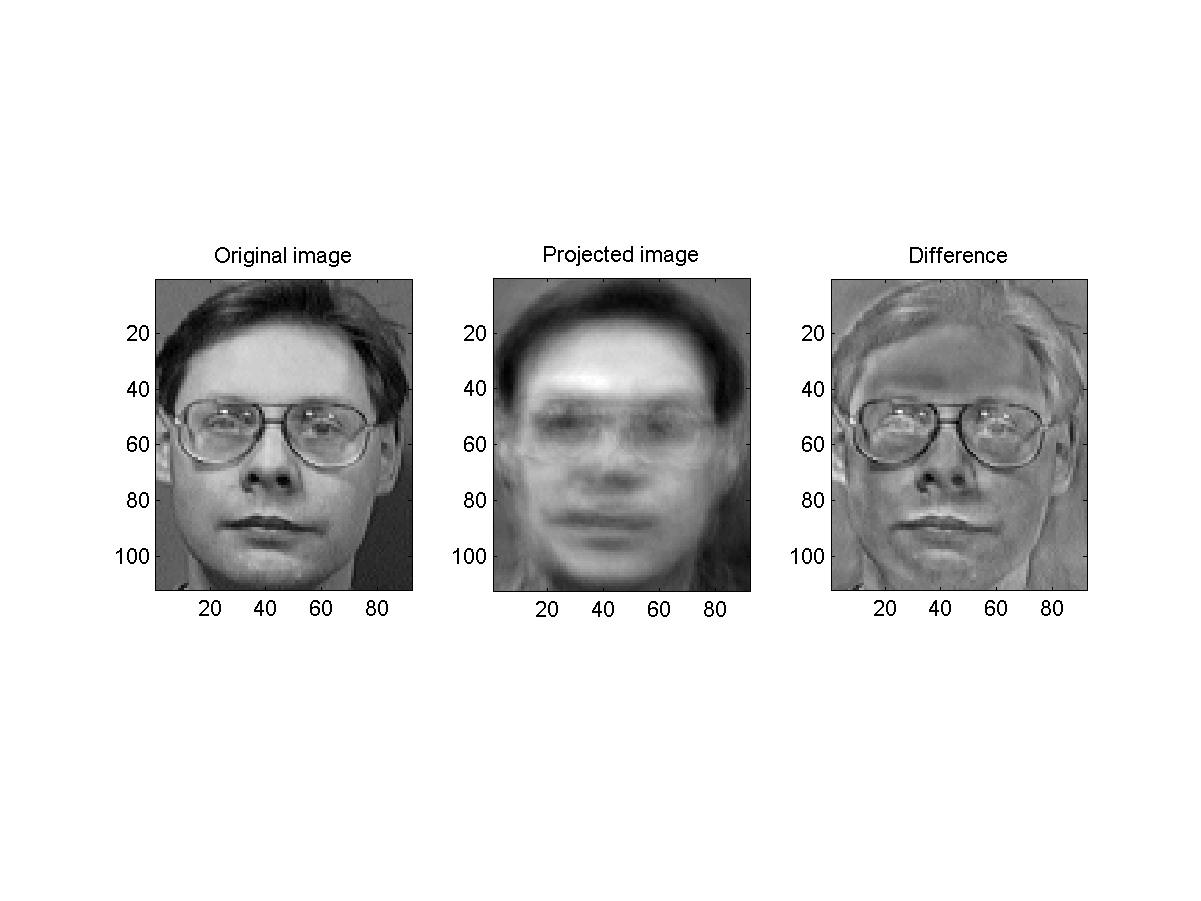On the other hand, if the original image is not a (human) face at all, then the difference between the original and projected images will be larger:

Example 14: faceRecog/facePcaProjDiff02.mload faceData.mat load eigenFaceResult.mat % Load A2, eigVec, cumVarPercent, rowDim, colDim eigVec2=eigVec(:, 1:28); % Take the first 28 eigenvectors origFace=double(imresize(imread('catPangPang.png'), [rowDim, colDim])); origFace=origFace(:); meanFace=mean(double(cat(3, faceData.image)), 3); meanFace=meanFace(:); projFace=eigVec2*(eigVec2'*(origFace-meanFace))+meanFace; subplot(1,3,1); imagesc(reshape(origFace, rowDim, colDim)); axis image; colormap(gray); title('Original image'); subplot(1,3,2); imagesc(reshape(projFace, rowDim, colDim)); axis image; colormap(gray); title('Projected image'); subplot(1,3,3); imagesc(reshape(origFace-projFace, rowDim, colDim)); axis image; colormap(gray); title('Difference'); fprintf('Difference between orig. and projected images = %g\n', norm(origFace-projFace)); Difference between orig. and projected images = 3008.69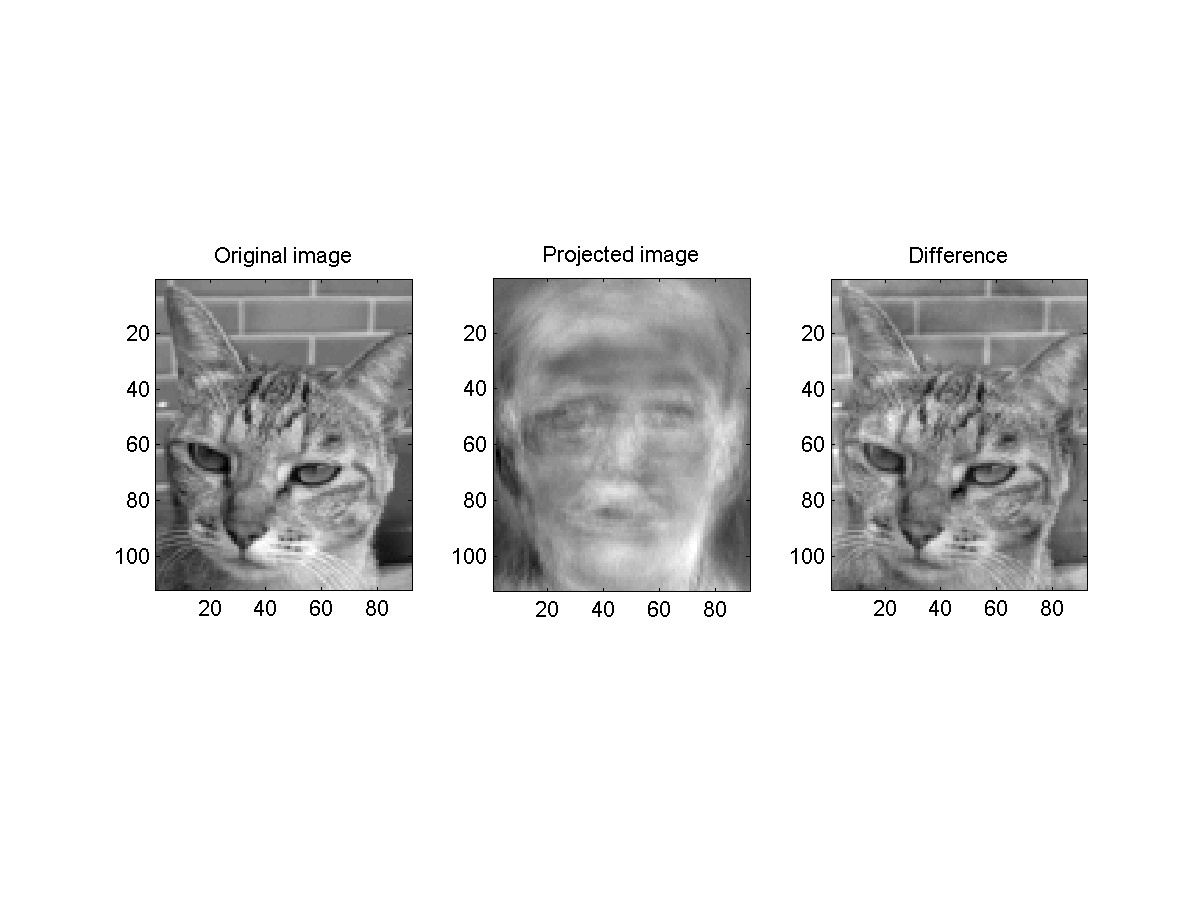Here the distance between the original face and the projected one is much larger than the one obtained in the previous example. As a result, it is possbile to use DFFS (distance from face space) to determine is a given image is a human face or not.

We can plot the histogram of the 400 DFFS, together with the faces that have the maximum and minimum DFFS:

Example 15: faceRecog/pcaDffsHist01.mload faceData.mat load eigenFaceResult.mat % Load A2, eigVec, cumVarPercent, rowDim, colDim meanFace=mean(double(cat(3, faceData.image)), 3); meanFace=meanFace(:); eigVec2=eigVec(:, 1:28); % Take the first 28 eigenvectors for i=1:length(faceData) temp=A2(29:end,i); dffs(i)=norm(temp); end subplot(3,1,1); hist(dffs, 30); [minValue, minIndex]=min(dffs); origFace=double(faceData(minIndex).image); origFace=origFace(:); projFace=eigVec2*(eigVec2'*(origFace-meanFace))+meanFace; subplot(3,3,4); imagesc(reshape(origFace, rowDim, colDim)); axis image; colormap(gray); title('Original image'); subplot(3,3,5); imagesc(reshape(projFace, rowDim, colDim)); axis image; colormap(gray); title('Projected image'); subplot(3,3,6); imagesc(reshape(origFace-projFace, rowDim, colDim)); axis image; colormap(gray); title('Difference'); fprintf('Min DFFS = %g\n', norm(origFace-projFace)); [maxValue, maxIndex]=max(dffs); origFace=double(faceData(maxIndex).image); origFace=origFace(:); projFace=eigVec2*(eigVec2'*(origFace-meanFace))+meanFace; subplot(3,3,7); imagesc(reshape(origFace, rowDim, colDim)); axis image; colormap(gray); title('Original image'); subplot(3,3,8); imagesc(reshape(projFace, rowDim, colDim)); axis image; colormap(gray); title('Projected image'); subplot(3,3,9); imagesc(reshape(origFace-projFace, rowDim, colDim)); axis image; colormap(gray); title('Difference'); fprintf('Max DFFS = %g\n', norm(origFace-projFace)); Min DFFS = 1353.94 Max DFFS = 2885.55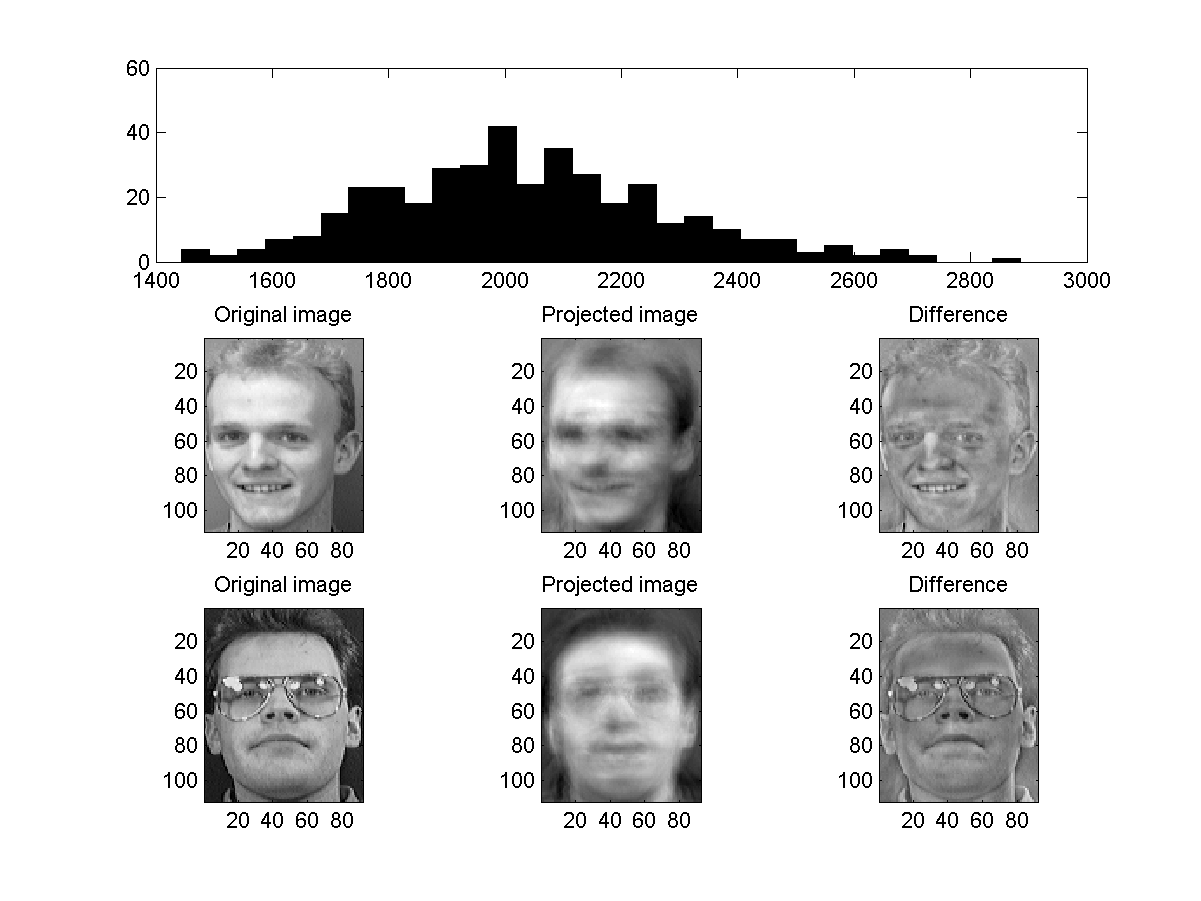For a give face, we can also find similar faces according to their distance within the face space:

Example 16: faceRecog/facePcaSimilarity01.mload faceData.mat load eigenFaceResult.mat % Load A2, eigVec, cumVarPercent, rowDim, colDim A2=A2(1:28, :); target=A2(:,1); allDistance=distPairwise(target, A2); personNum=length(faceData)/10; for i=1:personNum mask=inf*ones(length(allDistance), 1); index=(i-1)*10+1:i*10; mask(index)=allDistance(index); [distance(i), nearest(i)]=min(mask); end [minDistance, minIndex]=sort(distance); nearest=nearest(minIndex); nearest=reshape(nearest, personNum/4, 4)'; clear temp for i=1:4 temp(i).imageVec=[faceData(nearest(i,:)).image]; end imageMat=cat(1, temp.imageVec); subplot(2,1,1); imagesc(imageMat); axis image; colormap(gray); set(gca, 'xtick', []); set(gca, 'ytick', []); subplot(2,1,2); plot(minDistance, 'o-'); title('Distance (after projection) to the first image'); ylabel('Distance'); xlabel('Index of the sorted distance');